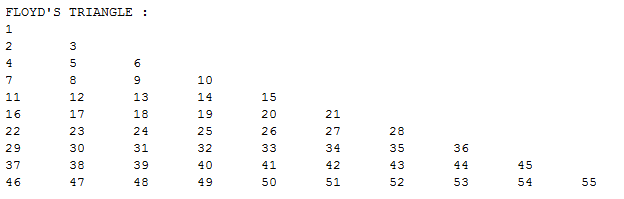## Tuesday, 20 January 2015

### Java Program to print Floyd's triangle

This is a simple Java Program which prints out the Floyd's triangle with respect to the input given.Java Program to print Floyd's triangle

PROGRAM :
```package codingcorner.in;

import java.util.Scanner;

public class FloydsTriangle {
public static void main(String[] args) {
int i, j, k = 1, n;
Scanner scan = new Scanner(System.in);
System.out.print("Enter the range: ");
n = scan.nextInt();
scan.close();
System.out.print("\nFLOYD'S TRIANGLE : \n");
for (i = 1; i <= n; i++) {
for (j = 1; j <= i; j++, k++) {
System.out.print(k + "\t");
}
System.out.print("\n");
}
}
}```

OUTPUT :Java Program to print Floyd's triangle